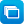Close message Scootle will stop supporting resources that use the Adobe Flash plug-in from 18 Dec 2020. Learning paths that include these resources will have alerts to notify teachers and students that one or more of the resources will be unavailable. Click here for more info.

# Mathematics / Year 2 / Number and Algebra / Number and place value

Curriculum content descriptions

Recognise and represent division as grouping into equal sets and solve simple problems using these representations (ACMNA032)

Elaborations
• dividing the class or a collection of objects into equal-sized groups
• identifying the difference between dividing a set of objects into three equal groups and dividing the same set of objects into groups of three
General capabilities
• Literacy Literacy
• Numeracy Numeracy
• Critical and creative thinking Critical and creative thinking
ScOT terms

Division,  Factors

## Refine results by

Year level F-2
Resource type
Learning area Mathematics

## Refine by topic

Related topic### Sites2See – number for primary

Selected links to a range of interactive online resources for the study of number in Foundation to Year 6 Mathematics.### What are factors?

What are factors? Watch as the jelly babies in this clip show you! What are the factors of 12? How many factors does the number 11 have? Try explaining to a friend what a prime number is.### Multiplication and division

Use these resources to develop your students' understanding and use of efficient strategies for multiplication and solving problems in authentic situations. Develop strategies for a sound basis of skip counting and other examples of repeated addition. Investigate division by sharing quantities (partition) then by repeated ...### reSolve: Authentic Problems: Bunches of Balloons

This sequence of three lessons introduces division and multiplication through the context of decorating a room with clusters of balloons. Students carry out an inquiry using a variety of processes associated with multiplication and division such as grouping concrete objects, arrays, repeated addition and skip counting. ...### Number line, by the Math Learning Centre - iTunes app

Use a virtual number line to visualise number sequences and to represent and solve addition, subtraction, multiplication and division problems. Free when reviewed on 12/5/2015.These seven learning activities, which focus on 'open-ended tasks' using a variety of tools (software) and devices (hardware), illustrate the ways in which content, pedagogy and technology can be successfully and effectively integrated in order to promote learning. In the activities, teachers use investigations in order ...### Mental calculation strategies - multiplication and division

This collection of 14 digital curriculum resources focuses on assisting students to develop efficient and effective mental calculation strategies for performing multiplication and division. The collection also aims to develop and consolidate students' knowledge of multiplicative number facts. It is organised in four categories: ...### Exploring division and multiplication

This collection of 17 digital curriculum resources contains activities to consolidate and extend students' problem-solving skills in the areas of multiplication and division. The first category, arrays, allows students to visualise problems while consolidating the idea that multiplication involves repeated groups of objects. ...### The array: go figure

This tutorial is suitable for use with a screen reader. It explains strategies for solving simple multiplications in your head such as 6x4. Work through sample questions and instructions explaining how to break up numbers into their factors. Solve multiplications by using arrays to break them up into rows and columns, then ...### Mental calculation strategies - addition and subtraction

This collection of 21 digital curriculum resources focuses on assisting students to develop efficient and effective mental calculation strategies for performing additions and subtractions of two-, three- and four-digit whole numbers. It is organised in three categories: developing strategies - addition; developing strategies ...### What is a quarter?

What is a quarter? You get quarters when you divide a whole into four equal parts. Each one of these four parts is a quarter. Watch this great explainer produced by Monique in collaboration with ABC Splash and see how she explains quarters.### Mental computation: basic facts - multiplication and division

This is a teacher resource that is part of a wider series; it develops a structured strategies-based approach to mental computation of single-digit multiplication and related division facts at lower primary level. It provides 22 engaging classroom activities that will stimulate students to use appropriate mental-computation ...### TIMES Module 3: Number and Algebra: multiplication and division - teacher guide

This is a 23-page guide for teachers. This module contains a description of suitable models for multiplication, a discussion of the type of problem phrased in words that requires multiplication for its solution, and mental and written strategies for multiplication. The use of the commutative, associative and distributive ...### reSolve: Multiplication - reSolve Fruit Shop

This sequence of two lessons explores the use of arrays to determine how many objects are in a collection. Students use strategies such as skip counting, repeated addition and partitioning the array into smaller parts. They investigate how some numbers can be represented as an array in different ways. They also explore ...### Multiply two numbers to get twenty!

Did you know that 5 times 4 equals 20? Did you also know that there are other numbers you can multiply to get to 20? See if you can come up with at least two other numbers.### Mental computation: introduction

This is a teacher resource that is part of a wider series; it introduces a structured strategies-based approach to mental computation for primary level and provides four generic classroom activities that can be used to empower students to use, share and test strategies. The support material describes the content of the ...### Multiples of five

When is a times table useful? Watch this video to see an example of when knowing a five times table comes in handy. Can you think of another example where knowing the times table could be useful?